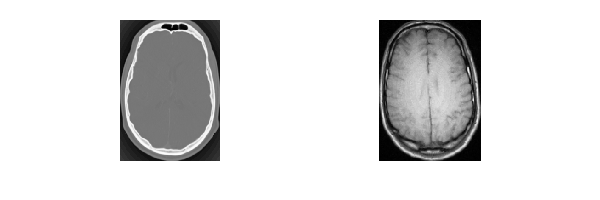# Data Augmentation for Deep Learning

Summary:

1. SimpleITK supports a variety of spatial transformations (global or local) that can be used to augment your dataset via resampling directly from the original images (which vary in size).
2. Resampling to a uniform size can be done either by specifying the desired sizes resulting in non-isotropic pixel spacings (most often) or by specifying an isotropic pixel spacing and one of the image sizes (width,height,depth).
3. SimpleITK supports a variety of intensity transformations (blurring, adding noise etc.) that can be used to augment your dataset after it has been resampled to the size expected by your network.

This notebook illustrates the use of SimpleITK to perform data augmentation for deep learning. Note that the code is written so that the relevant functions work for both 2D and 3D images without modification.

Data augmentation is a model based approach for enlarging your training set. The problem being addressed is that the original dataset is not sufficiently representative of the general population of images. As a consequence, if we only train on the original dataset the resulting network will not generalize well to the population (overfitting).

Using a model of the variations found in the general population and the existing dataset we generate additional images in the hope of capturing the population variability. Note that if the model you use is incorrect you can cause harm, you are generating observations that do not occur in the general population and are optimizing a function to fit them.

In :
import SimpleITK as sitk
import numpy as np
import os

%matplotlib nbagg
import gui

from utilities import parameter_space_regular_grid_sampling, similarity3D_parameter_space_regular_sampling, eul2quat

OUTPUT_DIR = 'output'


Load the images. You can work through the notebook using either the original 3D images or 2D slices from the original volumes.

In :
data = [sitk.ReadImage(fdata("training_001_ct.mha")),
# Comment out the following line if you want to work in 3D. Note that in 3D some of the notebook visualizations are
# disabled.
data = [data[:,:,data.GetDepth()//2], data[:,:,data.GetDepth()//2]]

Fetching training_001_ct.mha
Fetching training_001_mr_T1.mha

In :
def disp_images(images, fig_size, wl_list=None):
if images.GetDimension()==2:
gui.multi_image_display2D(image_list=images, figure_size=fig_size, window_level_list=wl_list)
else:
gui.MultiImageDisplay(image_list=images, figure_size=fig_size, window_level_list=wl_list)

disp_images(data, fig_size=(6,2))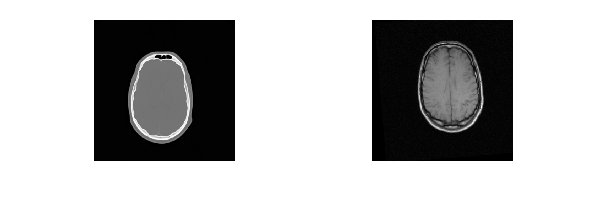The original data often needs to be modified. In this example we would like to crop the images so that we only keep the informative regions. We can readily separate the foreground and background using an appropriate threshold, in our case we use Otsu's threshold selection method.

In :
def threshold_based_crop_and_bg_median(image):
'''
Use Otsu's threshold estimator to separate background and foreground. In medical imaging the background is
usually air. Then crop the image using the foreground's axis aligned bounding box and compute the background
median intensity.
Args:
image (SimpleITK image): An image where the anatomy and background intensities form a bi-modal distribution
(the assumption underlying Otsu's method.)
Return:
Cropped image based on foreground's axis aligned bounding box.
Background median intensity value.
'''
# Set pixels that are in [min_intensity,otsu_threshold] to inside_value, values above otsu_threshold are
# set to outside_value. The anatomy has higher intensity values than the background, so it is outside.
inside_value = 0
outside_value = 255
bin_image = sitk.OtsuThreshold(image, inside_value, outside_value)

# Get the median background intensity
label_intensity_stats_filter = sitk.LabelIntensityStatisticsImageFilter()
label_intensity_stats_filter.SetBackgroundValue(outside_value)
label_intensity_stats_filter.Execute(bin_image,image)
bg_mean = label_intensity_stats_filter.GetMedian(inside_value)

# Get the bounding box of the anatomy
label_shape_filter = sitk.LabelShapeStatisticsImageFilter()
label_shape_filter.Execute(bin_image)
bounding_box = label_shape_filter.GetBoundingBox(outside_value)
# The bounding box's first "dim" entries are the starting index and last "dim" entries the size
return bg_mean, sitk.RegionOfInterest(image, bounding_box[int(len(bounding_box)/2):], bounding_box[0:int(len(bounding_box)/2)])

bg_means, modified_data = zip(*[threshold_based_crop_and_bg_median(img) for img in data])

disp_images(modified_data, fig_size=(6,2))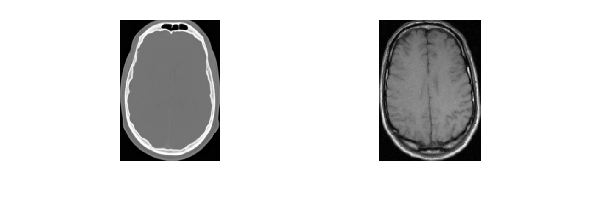At this point we select the images we want to work with, skip the following cell if you want to work with the original data.

In :
data = modified_data


# Augmentation using spatial transformations¶

We next illustrate the generation of images by specifying a list of transformation parameter values representing a sampling of the transformation's parameter space.

The code below is agnostic to the specific transformation and it is up to the user to specify a valid list of transformation parameters (correct number of parameters and correct order).

In most cases we can easily specify a regular grid in parameter space by specifying ranges of values for each of the parameters. In some cases specifying parameter values may be less intuitive (i.e. versor representation of rotation).

## Create reference domain¶

All input images will be resampled onto the reference domain.

This domain is defined by two constraints: the number of pixels per dimension and the physical size we want the reference domain to occupy. The former is associated with the computational constraints of deep learning where using a small number of pixels is desired. The later is associated with the SimpleITK concept of an image, it occupies a region in physical space which should be large enough to encompass the object of interest.

In :
dimension = data.GetDimension()

# Physical image size corresponds to the largest physical size in the training set, or any other arbitrary size.
reference_physical_size = np.zeros(dimension)
for img in data:
reference_physical_size[:] = [(sz-1)*spc if sz*spc>mx  else mx for sz,spc,mx in zip(img.GetSize(), img.GetSpacing(), reference_physical_size)]

# Create the reference image with a zero origin, identity direction cosine matrix and dimension
reference_origin = np.zeros(dimension)
reference_direction = np.identity(dimension).flatten()

# Select arbitrary number of pixels per dimension, smallest size that yields desired results
# or the required size of a pretrained network (e.g. VGG-16 224x224), transfer learning. This will
# often result in non-isotropic pixel spacing.
reference_size = *dimension
reference_spacing = [ phys_sz/(sz-1) for sz,phys_sz in zip(reference_size, reference_physical_size) ]

# Another possibility is that you want isotropic pixels, then you can specify the image size for one of
# the axes and the others are determined by this choice. Below we choose to set the x axis to 128 and the
# spacing set accordingly.
# Uncomment the following lines to use this strategy.
#reference_size_x = 128
#reference_spacing = [reference_physical_size/(reference_size_x-1)]*dimension
#reference_size = [int(phys_sz/(spc) + 1) for phys_sz,spc in zip(reference_physical_size, reference_spacing)]

reference_image = sitk.Image(reference_size, data.GetPixelIDValue())
reference_image.SetOrigin(reference_origin)
reference_image.SetSpacing(reference_spacing)
reference_image.SetDirection(reference_direction)

# Always use the TransformContinuousIndexToPhysicalPoint to compute an indexed point's physical coordinates as
# this takes into account size, spacing and direction cosines. For the vast majority of images the direction
# cosines are the identity matrix, but when this isn't the case simply multiplying the central index by the
# spacing will not yield the correct coordinates resulting in a long debugging session.
reference_center = np.array(reference_image.TransformContinuousIndexToPhysicalPoint(np.array(reference_image.GetSize())/2.0))


## Data generation¶

Once we have a reference domain we can augment the data using any of the SimpleITK global domain transformations. In this notebook we use a similarity transformation (the generate_images function is agnostic to this specific choice).

Note that you also need to create the labels for your augmented images. If these are just classes then your processing is minimal. If you are dealing with segmentation you will also need to transform the segmentation labels so that they match the transformed image. The following function easily accommodates for this, just provide the labeled image as input and use the sitk.sitkNearestNeighbor interpolator so that you do not introduce labels that were not in the original segmentation.

In :
def augment_images_spatial(original_image, reference_image, T0, T_aug, transformation_parameters,
output_prefix, output_suffix,
interpolator = sitk.sitkLinear, default_intensity_value = 0.0):
'''
Generate the resampled images based on the given transformations.
Args:
original_image (SimpleITK image): The image which we will resample and transform.
reference_image (SimpleITK image): The image onto which we will resample.
T0 (SimpleITK transform): Transformation which maps points from the reference image coordinate system
to the original_image coordinate system.
T_aug (SimpleITK transform): Map points from the reference_image coordinate system back onto itself using the
given transformation_parameters. The reason we use this transformation as a parameter
is to allow the user to set its center of rotation to something other than zero.
transformation_parameters (List of lists): parameter values which we use T_aug.SetParameters().
output_prefix (string): output file name prefix (file name: output_prefix_p1_p2_..pn_.output_suffix).
output_suffix (string): output file name suffix (file name: output_prefix_p1_p2_..pn_.output_suffix).
interpolator: One of the SimpleITK interpolators.
default_intensity_value: The value to return if a point is mapped outside the original_image domain.
'''
all_images = [] # Used only for display purposes in this notebook.
for current_parameters in transformation_parameters:
T_aug.SetParameters(current_parameters)
# Augmentation is done in the reference image space, so we first map the points from the reference image space
# back onto itself T_aug (e.g. rotate the reference image) and then we map to the original image space T0.
T_all = sitk.Transform(T0)
aug_image = sitk.Resample(original_image, reference_image, T_all,
interpolator, default_intensity_value)
sitk.WriteImage(aug_image, output_prefix + '_' +
'_'.join(str(param) for param in current_parameters) +'_.' + output_suffix)

all_images.append(aug_image) # Used only for display purposes in this notebook.
return all_images # Used only for display purposes in this notebook.


Before we can use the generate_images function we need to compute the transformation which will map points between the reference image and the current image as shown in the code cell below.

Note that it is very easy to generate large amounts of data, the calls to np.linspace with $m$ parameters each having $n$ values results in $n^m$ images, so don't forget that these images are also saved to disk. If you run the code below for 3D data you will generate 6561 volumes ($3^7$ parameter combinations times 3 volumes).

In :
if dimension == 2:
# The parameters are scale (+-10%), rotation angle (+-10 degrees), x translation, y translation
transformation_parameters_list = parameter_space_regular_grid_sampling(np.linspace(0.9,1.1,3),
np.linspace(-np.pi/18.0,np.pi/18.0,3),
np.linspace(-10,10,3),
np.linspace(-10,10,3))
aug_transform = sitk.Similarity2DTransform()
else:
transformation_parameters_list = similarity3D_parameter_space_regular_sampling(np.linspace(-np.pi/18.0,np.pi/18.0,3),
np.linspace(-np.pi/18.0,np.pi/18.0,3),
np.linspace(-np.pi/18.0,np.pi/18.0,3),
np.linspace(-10,10,3),
np.linspace(-10,10,3),
np.linspace(-10,10,3),
np.linspace(0.9,1.1,3))
aug_transform = sitk.Similarity3DTransform()

all_images = []
for index,img in enumerate(data):
# Transform which maps from the reference_image to the current img with the translation mapping the image
# origins to each other.
transform = sitk.AffineTransform(dimension)
transform.SetMatrix(img.GetDirection())
transform.SetTranslation(np.array(img.GetOrigin()) - reference_origin)
# Modify the transformation to align the centers of the original and reference image instead of their origins.
centering_transform = sitk.TranslationTransform(dimension)
img_center = np.array(img.TransformContinuousIndexToPhysicalPoint(np.array(img.GetSize())/2.0))
centering_transform.SetOffset(np.array(transform.GetInverse().TransformPoint(img_center) - reference_center))
centered_transform = sitk.Transform(transform)

# Set the augmenting transform's center so that rotation is around the image center.
aug_transform.SetCenter(reference_center)

generated_images = augment_images_spatial(img, reference_image, centered_transform,
aug_transform, transformation_parameters_list,
os.path.join(OUTPUT_DIR, 'spatial_aug'+str(index)),
'mha', default_intensity_value=bg_means[index])

if dimension==2: # in 2D we join all of the images into a 3D volume which we use for display.
all_images.append(sitk.JoinSeries(generated_images))
# If working in 2D, display the resulting set of images.
if dimension==2:
gui.MultiImageDisplay(image_list=all_images, shared_slider=True, figure_size=(6,2))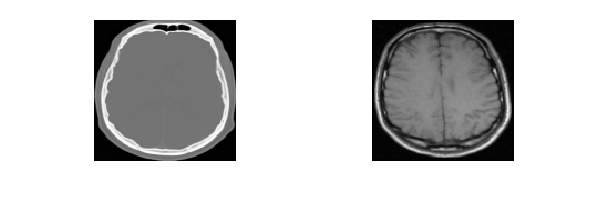Reflection using SimpleITK can be done in one of several ways:

1. Use an affine transform with the matrix component set to a reflection matrix. The columns of the matrix correspond to the $\mathbf{x}, \mathbf{y}$ and $\mathbf{z}$ axes. The reflection matrix is constructed using the plane, 3D, or axis, 2D, which we want to reflect through with the standard basis vectors, $\mathbf{e}_i, \mathbf{e}_j$, and the remaining basis vector set to $-\mathbf{e}_k$.
• Reflection about $xy$ plane: $[\mathbf{e}_1, \mathbf{e}_2, -\mathbf{e}_3]$.
• Reflection about $xz$ plane: $[\mathbf{e}_1, -\mathbf{e}_2, \mathbf{e}_3]$.
• Reflection about $yz$ plane: $[-\mathbf{e}_1, \mathbf{e}_2, \mathbf{e}_3]$.
2. Use the native slicing operator(e.g. img[:,::-1,:]), or the FlipImageFilter after the image is resampled onto the reference image grid.

We prefer option 1 as it is computationally more efficient. It combines all transformation prior to resampling, while the other approach performs resampling onto the reference image grid followed by the reflection operation. An additional difference is that using slicing or the FlipImageFilter will also modify the image origin while the resampling approach keeps the spatial location of the reference image origin intact. This minor difference is of no concern in deep learning as the content of the images is the same, but in SimpleITK two images are considered equivalent iff their content and spatial extent are the same.

The following cell corresponds to the preferred option, using an affine transformation:

In :
flipped_images = []
for img in data:
# Compute the transformation which maps between the reference and current image (same as done above).
transform = sitk.AffineTransform(dimension)
transform.SetMatrix(img.GetDirection())
transform.SetTranslation(np.array(img.GetOrigin()) - reference_origin)
centering_transform = sitk.TranslationTransform(dimension)
img_center = np.array(img.TransformContinuousIndexToPhysicalPoint(np.array(img.GetSize())/2.0))
centering_transform.SetOffset(np.array(transform.GetInverse().TransformPoint(img_center) - reference_center))
centered_transform = sitk.Transform(transform)

flipped_transform = sitk.AffineTransform(dimension)
flipped_transform.SetCenter(reference_image.TransformContinuousIndexToPhysicalPoint(np.array(reference_image.GetSize())/2.0))
if dimension==2: # matrices in SimpleITK specified in row major order
flipped_transform.SetMatrix([1,0,0,-1])
else:
flipped_transform.SetMatrix([1,0,0,0,-1,0,0,0,1])

# Resample onto the reference image
flipped_images.append(sitk.Resample(img, reference_image, centered_transform, sitk.sitkLinear, 0.0))
disp_images(flipped_images, fig_size=(6,2))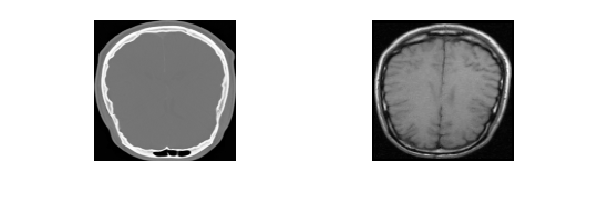Some 2D medical imaging modalities, such as endoscopic video and X-ray images acquired with C-arms using image intensifiers, exhibit radial distortion. The common model for such distortion was described by Brown ["Close-range camera calibration", Photogrammetric Engineering, 37(8):855–866, 1971]: $$\mathbf{p}_u = \mathbf{p}_d + (\mathbf{p}_d-\mathbf{p}_c)(k_1r^2 + k_2r^4 + k_3r^6 + \ldots)$$

where:

• $\mathbf{p}_u$ is a point in the undistorted image
• $\mathbf{p}_d$ is a point in the distorted image
• $\mathbf{p}_c$ is the center of distortion
• $r = \|\mathbf{p}_d-\mathbf{p}_c\|$
• $k_i$ are coefficients of the radial distortion

Using SimpleITK operators we represent this transformation using a deformation field as follows:

In :
def radial_distort(image, k1, k2, k3, default_intensity_value = 0.0, distortion_center=None):
c = distortion_center
if not c: # The default distortion center coincides with the image center
c = np.array(image.TransformContinuousIndexToPhysicalPoint(np.array(image.GetSize())/2.0))

# Compute the vector image (p_d - p_c)
delta_image = sitk.PhysicalPointSource( sitk.sitkVectorFloat64, image.GetSize(), image.GetOrigin(), image.GetSpacing(), image.GetDirection())
delta_image_list = [sitk.VectorIndexSelectionCast(delta_image,i) - c[i] for i in range(len(c))]

# Compute the radial distortion expression
r2_image = sitk.NaryAdd([img**2 for img in delta_image_list])
r4_image = r2_image**2
r6_image = r2_image*r4_image
disp_image = k1*r2_image + k2*r4_image + k3*r6_image
displacement_image = sitk.Compose([disp_image*img for img in delta_image_list])

displacement_field_transform = sitk.DisplacementFieldTransform(displacement_image)
return sitk.Resample(image, image, displacement_field_transform, sitk.sitkLinear,
default_intensity_value, image.GetPixelID())

k1 = 0.00001
k2 = 0.0000000000001
k3 = 0.0000000000001
original_ct_image = data
air_HU = -1000 #Hounsfield Unit value for air is -1000, background intensity for CT

#Using the default intensity value, zero, for background may not be the best choice when you have
#prior knowledge (e.g. we have a reasonable idea of what the background intensity value should be).
distorted_ct_image = radial_distort(original_ct_image, k1, k2, k3)
#distorted_ct_image = radial_distort(original_ct_image, k1, k2, k3, default_intensity_value = air_HU)

# Use a grid image to highlight the distortion.
grid_image = sitk.GridSource(outputPixelType=sitk.sitkUInt16, size=original_ct_image.GetSize(),
sigma=[0.1]*dimension, gridSpacing=[20.0]*dimension)
grid_image.CopyInformation(original_ct_image)
distorted_grid = radial_distort(grid_image, k1, k2, k3)
disp_images([original_ct_image, distorted_ct_image, distorted_grid], fig_size=(6,2))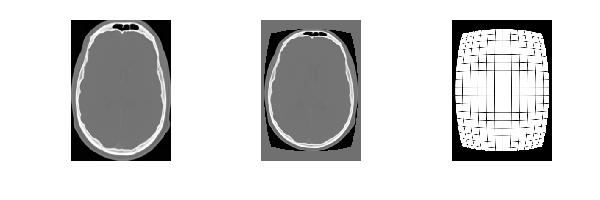### Transferring deformations - exercise for the interested reader¶

Using SimpleITK we can readily transfer deformations from a spatio-temporal data set to another spatial data set to simulate temporal behavior. Case in point, using a 4D (3D+time) CT of the thorax we can estimate the respiratory motion using non-rigid registration and Free Form Deformation or displacement field transformations. We can then register a new spatial data set to the original spatial CT (non-rigidly) followed by application of the temporal deformations.

Note that unlike the arbitrary spatial transformations we used for data-augmentation above this approach is more computationally expensive as it involves multiple non-rigid registrations. Also note that as the goal is to use the estimated transformations to create plausible deformations you may be able to relax the required registration accuracy.

# Augmentation using intensity modifications¶

SimpleITK has many filters that are potentially relevant for data augmentation via modification of intensities. For example:

In :
def augment_images_intensity(image_list, output_prefix, output_suffix):
'''
Generate intensity modified images from the originals.
Args:
image_list (iterable containing SimpleITK images): The images whose intensities we modify.
output_prefix (string): output file name prefix (file name: output_prefixi_FilterName.output_suffix).
output_suffix (string): output file name suffix (file name: output_prefixi_FilterName.output_suffix).
'''

# Create a list of intensity modifying filters, which we apply to the given images
filter_list = []

# Smoothing filters

filter_list.append(sitk.SmoothingRecursiveGaussianImageFilter())
filter_list[-1].SetSigma(2.0)

filter_list.append(sitk.DiscreteGaussianImageFilter())
filter_list[-1].SetVariance(4.0)

filter_list.append(sitk.BilateralImageFilter())
filter_list[-1].SetDomainSigma(4.0)
filter_list[-1].SetRangeSigma(8.0)

filter_list.append(sitk.MedianImageFilter())

# Noise filters using default settings

# Filter control via SetMean, SetStandardDeviation.

# Filter control via SetProbability
filter_list.append(sitk.SaltAndPepperNoiseImageFilter())

# Filter control via SetScale
filter_list.append(sitk.ShotNoiseImageFilter())

# Filter control via SetStandardDeviation
filter_list.append(sitk.SpeckleNoiseImageFilter())

filter_list[-1].SetAlpha(1.0)
filter_list[-1].SetBeta(0.0)

filter_list[-1].SetAlpha(0.0)
filter_list[-1].SetBeta(1.0)

aug_image_lists = [] # Used only for display purposes in this notebook.
for i,img in enumerate(image_list):
aug_image_lists.append([f.Execute(img) for f in filter_list])
for aug_image,f in zip(aug_image_lists[-1], filter_list):
sitk.WriteImage(aug_image, output_prefix + str(i) + '_' +
f.GetName() + '.' + output_suffix)
return aug_image_lists


Modify the intensities of the original images using the set of SimpleITK filters described above. If we are working with 2D images the results will be displayed inline.

In :
intensity_augmented_images = augment_images_intensity(data, os.path.join(OUTPUT_DIR, 'intensity_aug'), 'mha')

# in 2D we join all of the images into a 3D volume which we use for display.
if dimension==2:
def list2_float_volume(image_list) :
return sitk.JoinSeries([sitk.Cast(img, sitk.sitkFloat32) for img in image_list])

all_images = [list2_float_volume(imgs) for imgs in intensity_augmented_images]

# Compute reasonable window-level values for display (just use the range of intensity values
# from the original data).
original_window_level = []
statistics_image_filter = sitk.StatisticsImageFilter()
for img in data:
statistics_image_filter.Execute(img)
max_intensity = statistics_image_filter.GetMaximum()
min_intensity = statistics_image_filter.GetMinimum()
original_window_level.append((max_intensity-min_intensity, (max_intensity+min_intensity)/2.0))
gui.MultiImageDisplay(image_list=all_images, shared_slider=True, figure_size=(6,2), window_level_list=original_window_level)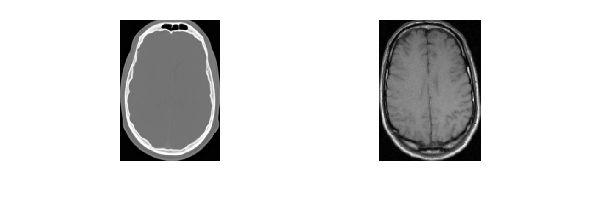Finally, you can easily create intensity variations that are specific to your domain, such as the spatially varying multiplicative and additive transformation shown below.

In :
def mult_and_add_intensity_fields(original_image):
'''
Modify the intensities using multiplicative and additive Gaussian bias fields.
'''
# Gaussian image with same meta-information as original (size, spacing, direction cosine)
# Sigma is half the image's physical size and mean is the center of the image.
g_mult = sitk.GaussianSource(original_image.GetPixelIDValue(),
original_image.GetSize(),
[(sz-1)*spc/2.0 for sz, spc in zip(original_image.GetSize(), original_image.GetSpacing())],
original_image.TransformContinuousIndexToPhysicalPoint(np.array(original_image.GetSize())/2.0),
25,
original_image.GetOrigin(),
original_image.GetSpacing(),
original_image.GetDirection())

# Gaussian image with same meta-information as original (size, spacing, direction cosine)
# Sigma is 1/8 the image's physical size and mean is at 1/16 of the size
original_image.GetSize(),
[(sz-1)*spc/8.0 for sz, spc in zip(original_image.GetSize(), original_image.GetSpacing())],
original_image.TransformContinuousIndexToPhysicalPoint(np.array(original_image.GetSize())/16.0),
25,
original_image.GetOrigin(),
original_image.GetSpacing(),
original_image.GetDirection())